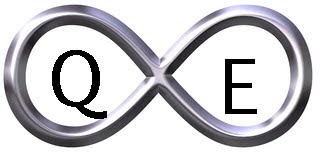### AmenRa's Corner 5/22/15Creditcane™: REPETERE AD INFINITUM: CAVEAT EMPTOR.

SPY (SPDR S&P 500)
new high 212.99
trend=up
high= 212.99
rev= 211.24; mid= 212.12

monthly info -no change (above mid)
trend=up
high= 210.66; rev= 200.71; mid= 205.69

XLB (SPDR Materials)
-no change (above mid)
trend=up
high= 51.93
rev= 48.44; mid= 50.19

monthly info -change (above mid)
trend=no; direction=down (1 bar)
low= 48.78; rev= 51.49; mid= 50.14

XLE (SPDR Energy)
-no change (above mid)
trend=down
low= 74.02
rev= 83.55; mid= 78.79

monthly info -change (above mid)
trend=down
low= 75.55; rev= 87.42; mid= 81.49

XLF (SPDR Financial)
new high 24.86
trend=up
high= 24.86
rev= 24.44; mid= 24.65

monthly info -no change (above mid)
trend=no; direction=down (1 bar)
low= 23.01; rev= 24.73; mid= 23.87

XLI (SPDR Industrials)
-no change (above mid)
trend=no
direction=up (1 bar)
high= 58.16
rev= 54.57; mid= 56.37

monthly info -change (below mid)
trend=up
high= 57.49; rev= 54.05; mid= 55.77

XLK (SPDR Technology)
new high 43.60
trend=up
high= 43.60
rev= 43.09; mid= 43.35

monthly info -change (above mid)
trend=up
high= 43.09; rev= 40.28; mid= 41.69

XLP (SPDR Consumer Staples)
-no change (above mid)
trend=no
direction=up (1 bar)
high= 50.01
rev= 48.02; mid= 49.02

monthly info -no change (above mid)
trend=up
high= 50.01; rev= 45.13; mid= 47.57

XLU (SPDR Utilities)
-no change (below mid)
trend=down
low= 43.39
rev= 48.62; mid= 46.01

monthly info MONTHLY CONFIRMATION new low 44.22
trend=down
low= 44.22; rev= 47.22; mid= 45.72

XLV (SPDR Healthcare)
new high 74.98
trend=up
high= 74.98
rev= 72.18; mid= 73.58

monthly info -no change (above mid)
trend=up
high= 72.50; rev= 67.27; mid= 69.89

XLY (SPDR Consumer Discretionary)
-no change (above mid)
trend=up
high= 77.63
rev= 75.44; mid= 76.54

monthly info -no change (above mid)
trend=up
high= 75.97; rev= 68.82; mid= 72.40

XPH (SPDR Pharmaceuticals)
-change (above mid)
trend=down
low= 119.93
rev= 123.41; mid= 121.67

monthly info -no change (above mid)
trend=up
high= 123.68; rev= 106.01; mid= 114.85

XRT (SPDR Retail)
-no change (below mid)
trend=no
direction=down (2 bars)
low= 98.20
rev= 101.44; mid= 99.82

monthly info -change (below mid)
trend=up
high= 101.04; rev= 94.31; mid= 97.68

RBOB (RBOB Gasoline)
-no change (below mid)
trend=up
high= 3.57
rev= 1.51; mid= 2.54

monthly info -no change (below mid)
trend=no; direction=up (2 bars)
high= 3.54; rev= 1.57; mid= 2.56IS IT OVER? IS IT REALLY OVER?

AmenRa said...

Weekly 3LB Update 5/22/15

AmenRa said...

New.